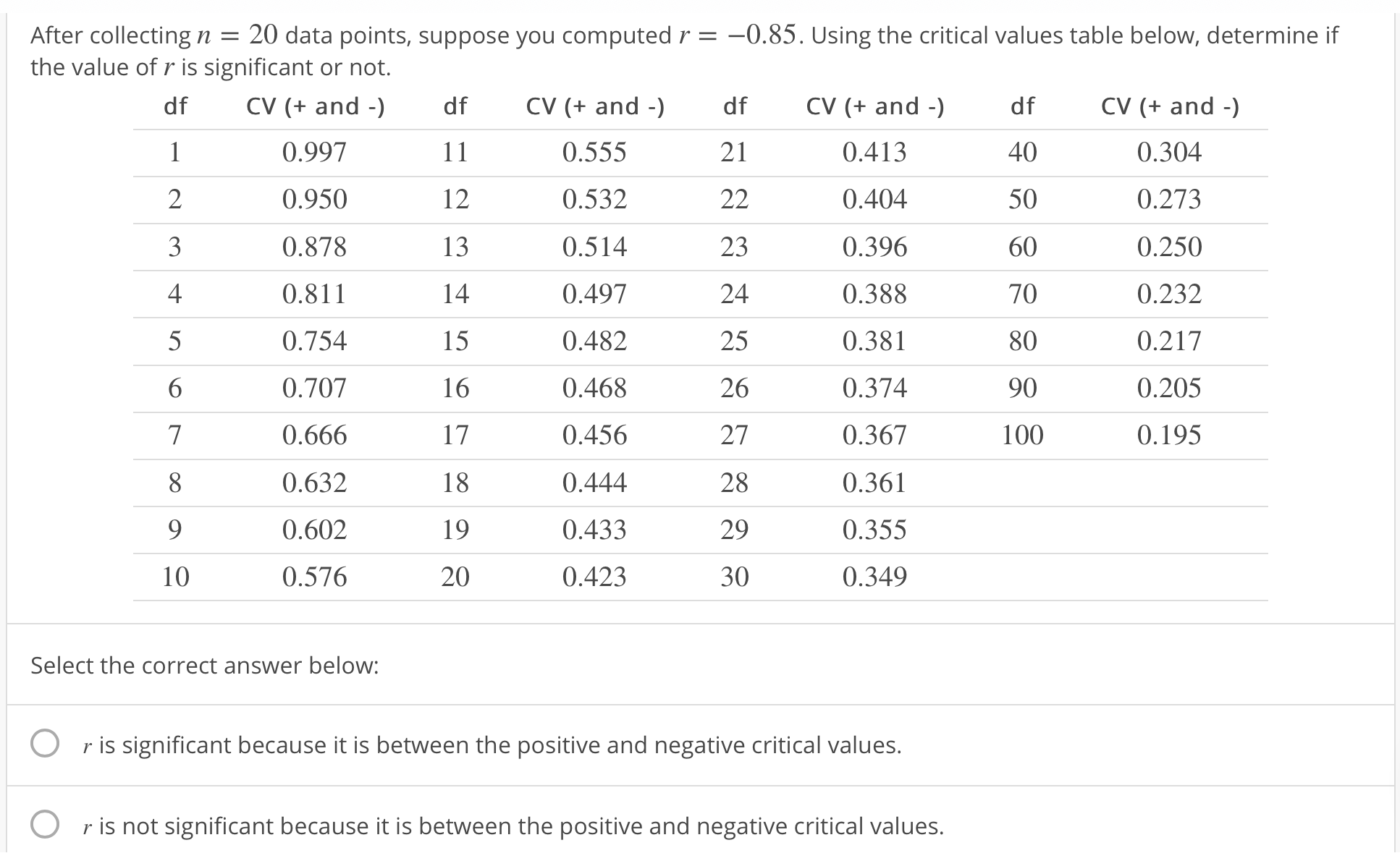# After collecting n = 20 data points, suppose you computed rthe value of r is significant or not.-0.85. Using the critical values table below, determine ifdf CV (+ and0.9970.9500.8780.8110.7540.7070.6660.6320.6020.576df CV (+ and - dfCV (+ and -)df405060708090100CV (+ and -)0.3040.2730.2500.2320.2170.2050.1950.5550.5320.5140.4970.4820.4680.4560.4440.4330.4230.4130.4040.3960.3880.3810.3740.3670.3610.3550.34923423242526272829301315161718192010Select the correct answer below:Or is significant because it is between the positive and negative critical valuesr is not significant because it is between the positive and negative critical values.

Question
48 views

r is significant because it is between the positive and negative critical values.

r is not significant because it is between the positive and negative critical values.

r is significant because it is not between the positive and negative critical values.

r is not significant because it is not between the positive and negative critical values.help_outlineImage TranscriptioncloseAfter collecting n = 20 data points, suppose you computed r the value of r is significant or not. -0.85. Using the critical values table below, determine if df CV (+ and 0.997 0.950 0.878 0.811 0.754 0.707 0.666 0.632 0.602 0.576 df CV (+ and - dfCV (+ and -) df 40 50 60 70 80 90 100 CV (+ and -) 0.304 0.273 0.250 0.232 0.217 0.205 0.195 0.555 0.532 0.514 0.497 0.482 0.468 0.456 0.444 0.433 0.423 0.413 0.404 0.396 0.388 0.381 0.374 0.367 0.361 0.355 0.349 2 3 4 23 24 25 26 27 28 29 30 13 15 16 17 18 19 20 10 Select the correct answer below: O r is significant because it is between the positive and negative critical values r is not significant because it is between the positive and negative critical values. fullscreen
check_circle

star
star
star
star
star
1 Rating
Step 1

From the given information, the sample size n = 20.

Therefore, the degrees of freedom is n – 2 = 20-2 = 18.

The correlation coefficient is -0.85.

From the given table, corresponding to the 18 degrees of freedom, the critical value is ±0.444.

Step 2

Rule for the significant r:

• If the correlation coefficient, r is not between the two critical values that is not between the negative and positive critical values ,r is significant.
• Otherwise, r is insignificant.

Here, -0.85<-0.444.

That is, the correlation coefficient is not between the two critical values, -0.444 and 0.444.

Therefore, r is significant.

It can be shown as below,

Step 3

Therefore, the third option is correct that r is significant because it is not between the positive and negative critical values.

1st option is contradictory of 3rd option, hence, 1s option i...

### Want to see the full answer?

See Solution

#### Want to see this answer and more?

Solutions are written by subject experts who are available 24/7. Questions are typically answered within 1 hour.*

See Solution
*Response times may vary by subject and question.
Tagged in

### Other